Test: Electric Field due to Continuous Charge Distribution

# Test: Electric Field due to Continuous Charge Distribution

Test Description

## 5 Questions MCQ Test Electromagnetic Fields Theory (EMFT) | Test: Electric Field due to Continuous Charge Distribution

Test: Electric Field due to Continuous Charge Distribution for Electrical Engineering (EE) 2023 is part of Electromagnetic Fields Theory (EMFT) preparation. The Test: Electric Field due to Continuous Charge Distribution questions and answers have been prepared according to the Electrical Engineering (EE) exam syllabus.The Test: Electric Field due to Continuous Charge Distribution MCQs are made for Electrical Engineering (EE) 2023 Exam. Find important definitions, questions, notes, meanings, examples, exercises, MCQs and online tests for Test: Electric Field due to Continuous Charge Distribution below.
Solutions of Test: Electric Field due to Continuous Charge Distribution questions in English are available as part of our Electromagnetic Fields Theory (EMFT) for Electrical Engineering (EE) & Test: Electric Field due to Continuous Charge Distribution solutions in Hindi for Electromagnetic Fields Theory (EMFT) course. Download more important topics, notes, lectures and mock test series for Electrical Engineering (EE) Exam by signing up for free. Attempt Test: Electric Field due to Continuous Charge Distribution | 5 questions in 15 minutes | Mock test for Electrical Engineering (EE) preparation | Free important questions MCQ to study Electromagnetic Fields Theory (EMFT) for Electrical Engineering (EE) Exam | Download free PDF with solutions
 1 Crore+ students have signed up on EduRev. Have you?
Test: Electric Field due to Continuous Charge Distribution - Question 1

### An infinitely long uniform charge of density 30 nC/m is located at y = 3, z = 4. The field intensity at (0, 5, 1) is E. Now, what is the field intensity at (2, 5, 1)?

Detailed Solution for Test: Electric Field due to Continuous Charge Distribution - Question 1

Concept:
The magnitude of electric field intensity at a point P due to infinitely long uniform charge density λ is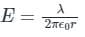r → the perpendicular distance from the point to the infinitely long charge density
Analysis:
Here, the point (0, 5, 1) and (2, 5, 1)
r is the same and is equal to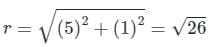∴ the electric field will be same in both the cases.

Test: Electric Field due to Continuous Charge Distribution - Question 2

### The electric flux lines

Detailed Solution for Test: Electric Field due to Continuous Charge Distribution - Question 2

Electric flux lines originate from positive charges and terminate at negative charges as shown:​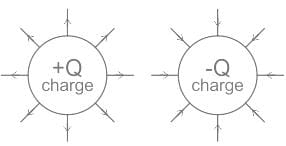Note:
It is the magnetic field lines that form a closed loop as shown: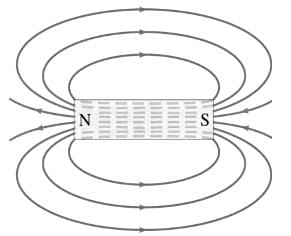Test: Electric Field due to Continuous Charge Distribution - Question 3

### The electric field E at (1, 1, -1) due to three charge distributions – 10nC/m2 at plane x = 2, 15 nC/m2 at plane y = -3 and 10 π nc/m line charge at x = 0, z = 2 is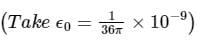Detailed Solution for Test: Electric Field due to Continuous Charge Distribution - Question 3

The electric charge distributions are as shown below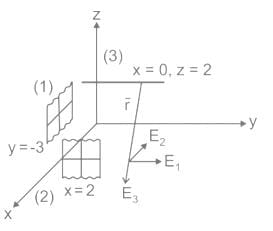The filed due to plane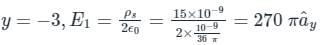The field due to plane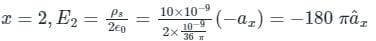The field due to line charge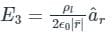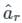is the unit vector along perpendicular to the line charge passing through P.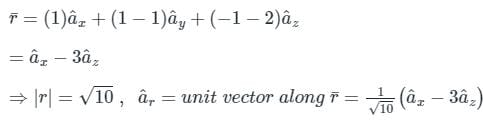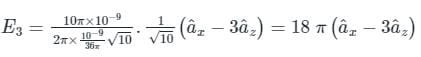∴ Total electric field E = E1 + E2 + E3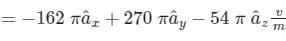*Answer can only contain numeric values
Test: Electric Field due to Continuous Charge Distribution - Question 4

The electric field at a distance of 2 m from an infinite line charge of charge density 4 nC/m is ______V/m.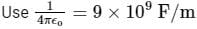Detailed Solution for Test: Electric Field due to Continuous Charge Distribution - Question 4

The electric due to an infinite line charge is given by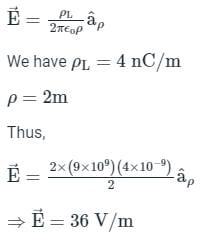Test: Electric Field due to Continuous Charge Distribution - Question 5

Let  Q1 = 4μ C be located at P1(3,11,8) while Q2 = -5μC is at P2(6,15,8).The force F­2 on Q2 will be

Detailed Solution for Test: Electric Field due to Continuous Charge Distribution - Question 5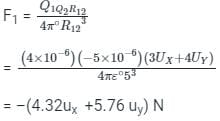## Electromagnetic Fields Theory (EMFT)

11 videos|46 docs|62 tests
 Use Code STAYHOME200 and get INR 200 additional OFF Use Coupon Code
Information about Test: Electric Field due to Continuous Charge Distribution Page
In this test you can find the Exam questions for Test: Electric Field due to Continuous Charge Distribution solved & explained in the simplest way possible. Besides giving Questions and answers for Test: Electric Field due to Continuous Charge Distribution, EduRev gives you an ample number of Online tests for practice

## Electromagnetic Fields Theory (EMFT)

11 videos|46 docs|62 tests